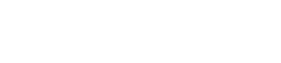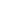## Mode Studio

The Collaborative Data Science Platform# SQL GROUP BY

Starting here? This lesson is part of a full-length tutorial in using SQL for Data Analysis. Check out the beginning.

## The SQL GROUP BY clause

SQL aggregate function like `COUNT`, `AVG`, and `SUM` have something in common: they all aggregate across the entire table. But what if you want to aggregate only part of a table? For example, you might want to count the number of entries for each year.

In situations like this, you'd need to use the `GROUP BY` clause. `GROUP BY` allows you to separate data into groups, which can be aggregated independently of one another. Here's an example using the Apple stock prices dataset:

``````SELECT year,
COUNT(*) AS count
FROM tutorial.aapl_historical_stock_price
GROUP BY year
``````

You can group by multiple columns, but you have to separate column names with commas—just as with `ORDER BY`):

``````SELECT year,
month,
COUNT(*) AS count
FROM tutorial.aapl_historical_stock_price
GROUP BY year, month
``````

## Practice Problem

Calculate the total number of shares traded each month. Order your results chronologically.

Try it out See the answer

## GROUP BY column numbers

As with `ORDER BY`, you can substitute numbers for column names in the `GROUP BY` clause. It's generally recommended to do this only when you're grouping many columns, or if something else is causing the text in the `GROUP BY` clause to be excessively long:

``````SELECT year,
month,
COUNT(*) AS count
FROM tutorial.aapl_historical_stock_price
GROUP BY 1, 2
``````

Note: this functionality (numbering columns instead of using names) is supported by Mode, but not by every flavor of SQL, so if you're using another system or connected to certain types of databases, it may not work.

## Using GROUP BY with ORDER BY

The order of column names in your `GROUP BY` clause doesn't matter—the results will be the same regardless. If you want to control how the aggregations are grouped together, use `ORDER BY`. Try running the query below, then reverse the column names in the `ORDER BY` statement and see how it looks:

``````SELECT year,
month,
COUNT(*) AS count
FROM tutorial.aapl_historical_stock_price
GROUP BY year, month
ORDER BY month, year
``````

## Using GROUP BY with LIMIT

There's one thing to be aware of as you group by multiple columns: SQL evaluates the aggregations before the `LIMIT` clause. If you don't group by any columns, you'll get a 1-row result—no problem there. If you group by a column with enough unique values that it exceeds the `LIMIT` number, the aggregates will be calculated, and then some rows will simply be omitted from the results.

This is actually a nice way to do things because you know you're going to get the correct aggregates. If SQL cuts the table down to 100 rows, then performed the aggregations, your results would be substantially different. The above query's results exceed 100 rows, so it's a perfect example. Try removing the limit and running it again to see what changes.

## Practice Problem

Write a query to calculate the average daily price change in Apple stock, grouped by year.

Try it out See the answer

## Practice Problem

Write a query that calculates the lowest and highest prices that Apple stock achieved each month.

Try it out See the answer

Next Lesson

SQL HAVING

Get the latest.